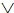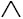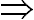# Mathematical Logic QuestionsWhich of the following is not a statement
(a) Every set is a finite set
(b) 8 is less than 6
(c) Where are you going ?
(d) The sum of interior angles of a triangle is 180 degrees

Ans. (c)

Which of the following is not a statement
(a) Please do me a favour
(b) 2 is an even integer
(c) 2 + 1 = 3
(d) The number 17 is prime

Ans. (a)

Which of the following is an open statement
(a) x is a natural number
(b) Give me a glass of water
(c) Wish you best of luck
(d) Good morning to all

Ans. (a)

If p, q, r are simple propositions with truth values T, F, T, then the truth value of (~ pq)~ rp is
(a)

True
(b) False
(c) True if r is false
(d) True if q is true

Ans. (a)

If (p~ r)(qr) is false and q and r are both false, then p is
(a) True
(b) False
(c) May be true or false
(d) Data insufficient

Ans. (a)

(p~ q)(~ pq) is
(b) A tautology
(c) Either (a) or (b)
(d) Neither (a) nor (b)

Ans. (a)

Which of the following is not logically equivalent to the proposition : “A real number is either rational or irrational”.
(a) If a number is neither rational nor irrational then it is not real
(b) If a number is not a rational or not an irrational, then it is not real
(c) If a number is not real, then it is neither rational nor irrational
(d) If a number is real, then it is rational or irrational

Ans. (b)

If p : It rains today, q : I go to school, r : I shall meet any friends and s : I shall go for a movie, then which of the following is the proposition :
If it does not rain or if I do not go to school, then I shall meet my friend and go for a movie.
(a) ~ (pq) -> (rs)
(b) ~ (p~ q) -> (rs)
(c) ~ (pq) -> (rs)
(d) None of these

Ans. (a)

Related: mathematical induction questions

The propositions (p~ p)(~ pp) is a
(d) Tautology

Ans. (c)

If p(qr) is false, then the truth values of p, q, r are respectively
(a) T, F, F
(b) F, F, F
(c) F, T, T
(d) T, T, F

Ans. (a)

If p(~ pq) is false, the truth values of p and q are respectively
(a) F, T
(b) F, F
(c) T, T
(d) T, F
Ans. (d)

Related: questions on carboxylic acids

(p~ q)(~ pq) is
(a) A tautology
(c) Both a tautology and a contradiction
(d) Neither a tautology nor a contradiction

Ans. (b)

Which of the following is the inverse of the proposition : “If a number is a prime then it is odd.”
(a) If a number is not a prime then it is odd
(b) If a number is not a prime then it is odd
(c) If a number is not odd then it is not a prime
(d) If a number is not odd then it is a prime

Ans. (b)

~ (p(~q)) is equal to
(a) ~ pq
(b) (~p)q
(c) ~ p~ p
(d) ~p~ q

Ans. (b)

Which of the following is logically equivalent to ~ (~ pq)
(a) pq
(b) p~ q
(c) ~ pq
(d) ~ p~ q
Ans. (d)

The conditional (pq)p is
(a) A tautology